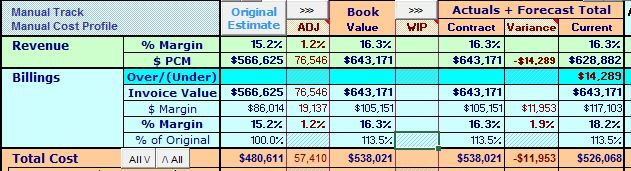# Summary module with multiple calculated measuresHi All ,

I am trying to make a summary module which has mutiple calculated measures . Summary module is like an intersection between these calculated measures . Eg belowon

vertical axis i have measures like revenue \$ revenue margin % to cost invoice cost etc

on horizontal axis i have measures like original value , adjustments book value ( original value + adj) WIP  , contrcat value ( book value + WIP ) , current ( Actuals + forecast ) and variance ( contract - current value )

I am confused with what would become my lists and what would become by line items .?

Any help will be really appreciated

Tagged:

•@karank to give a quick answer, the rule of thumb is that all things that need to be calculated have to be a line item. In your case, it is both rows and columns. The 1st column is just a grouping which you could just combine with the 2nd column. Once you get the values in the rows calculated, you can create a Line Item Subset and then create a new module using the newly created Line Item Subset and the line items would be the ones shown in the column and 1 more line item with COLLECT() formula.

This might be too high level but you would need at least two modules where one is calculating the measures shown on the rows then the second one to display the items separately and perhaps do additional calculations on top of the 1st module.

Hope it is helpful. Let me know if this works.

Thanks,

Leo